• 后序遍历输出
2020-05-07 12:33:36

# 由中序遍历和后序遍历 输出先序遍历

public static void main(String[] args) {
Scanner input=new Scanner(System.in);
String mid=input.nextLine();
String next=input.nextLine();
System.out.println(change(mid,next));
}

private static String change(String mid, String next) {
if(mid.length()>0){
int len=next.length();
if(len == 1)
return next;
if(len <= 0 ||len > 8)
return "";
char root=next.charAt(len - 1);
int rootIndex = mid.indexOf(root);
return next.charAt(len - 1) + change(mid.substring(0,rootIndex),next.substring(0,rootIndex)) + change(mid.substring(rootIndex+1),next.substring(rootIndex,len - 1));
}else
return "";
}java
更多相关内容
• [问题描述] 建立二叉树，并输出二叉树的先序，中序和后序遍历序列，以及二叉树的叶子数。 [基本要求] 要求根据读取的元素建立二叉树，能输出各种遍历。 [实现提示] 可通过输入带空格的前序序列建立二叉链表。
• NULL 博文链接：https://128kj.iteye.com/blog/1692248
• 题目：建立一棵用二叉链表方式存储的二叉树，并对其进行遍历（先序、中序和后序），将遍历序列输出到显示器上。 【基本要求】从键盘输入一棵... printf("\n输出后序遍历："); PostOrder(bt); return 0; } 运行结果：

题目：建立一棵用二叉链表方式存储的二叉树，并对其进行遍历（先序、中序和后序），将遍历序列输出到显示器上。

【基本要求】从键盘输入一棵二叉树的先序序列，以二叉链表作为存储结构，建立二叉树并对其进行遍历（先序、中序和后序），然后将遍历结果打印输出。

代码如下：

#include<stdio.h>
#include<stdlib.h>
//定义二叉树链表结点结构
typedef struct Node
{
char data;
struct Node* Lchild;
struct Node* Rchild;
}BiTree, * PBiTree;

//创建二叉树
void CreateBiTree(PBiTree* bt)    //此时bt为二级指针
{
char ch;
ch = getchar();
if (ch == '*')
{
*bt = NULL;
}
else
{
*bt = (PBiTree)malloc(sizeof(BiTree));
(*bt)->data = ch;
CreateBiTree(&((*bt)->Lchild));   //一级指针值为空，二级指针要把地址传入
CreateBiTree(&((*bt)->Rchild));
}
}
//先序遍历
void PreOrder(PBiTree root)
{
if (root != NULL)
{
printf("%c", root->data);
PreOrder(root->Lchild);
PreOrder(root->Rchild);
}
}
//中序遍历
void InOrder(BiTree* root)
{
if (root != NULL)
{
InOrder(root->Lchild);
printf("%c", root->data);
InOrder(root->Rchild);
}
}
//后序遍历
void PostOrder(BiTree* root)
{
if (root != NULL)
{
PostOrder(root->Lchild);
PostOrder(root->Rchild);
printf("%c", root->data);
}
}

int main()
{
PBiTree bt = NULL;//创建一个空树
printf("输入先序二叉树结点(子树为空输入*)：\n");
CreateBiTree(&bt);
printf("\n输出先序遍历：");
PreOrder(bt);
printf("\n输出中序遍历：");
InOrder(bt);
printf("\n输出后序遍历：");
PostOrder(bt);
return 0;
}


运行结果：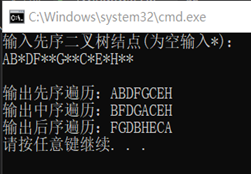展开全文数据结构 二叉树
• 根据树的前序遍历和中序遍历构造树并输出后序遍历代码如下： <?php class BinaryTreeNode{ public $m_value; public$m_left; public $m_right; } function ConstructCore($preorder,$inorder){ if(count($...
• C语言实现数据结构中根据中序、后序遍历构建树，并进行先序与层次遍历，以树形结构输出，附有详细中文注释
• 本文实例讲述了Python实现输入二叉树的先序和中序遍历，再输出后序遍历操作。分享给大家供大家参考，具体如下： 实现一个功能：  输入:一颗二叉树的先序和中序遍历  输出:后续遍历 思想： 先序遍历中，第一个元素...
• 回想由中序遍历和后序遍历确定二叉树，我们每次都找下一个更小的左右子树范围的区间进行递归。 那么这个思路延续。根据完全二叉树来先递归建立好空树，在建立过程中push_up每个节点子树的siz. 然后在输入的数组中...

思路：有个很关键的条件就是树的大小能定下来，而且是按照一个个顺序放的。

回想由中序遍历和后序遍历确定二叉树，我们每次都找下一个更小的左右子树范围的区间进行递归。

那么这个思路延续。根据完全二叉树来先递归建立好空树，在建立过程中push_up每个节点子树的siz.

然后在输入的数组中进行递归找到子树，最后用bfs输出一下就好。

感觉是最近线段树主席树做魔怔了，上来就套。但是如果去模拟就知道，线段树和这个树完全对应的情况只有满树的时候，不然建出来的树对应的状态是不同的。

因为线段树是由区间来划分的，而这些课上的树是节点来划分的。

注意：代码中递归的边界都是用左子树当前来算的。（这个bug我调了好久

#include<iostream>
#include<vector>
#include<queue>
#include<cstring>
#include<cmath>
#include<map>
#include<set>
#include<cstdio>
#include<algorithm>
#define debug(a) cout<<#a<<"="<<a<<endl;
using namespace std;
const int maxn=1e5;
typedef int LL;
LL n;
struct Tree{
LL lson,rson;
LL val;
LL siz;
}tree[maxn*4];
LL a[maxn];
LL build(LL p){///先建好对应的空树
if(p>n) {
tree[p].siz=0;
return -1;
}
tree[p].siz=1;
tree[p].val=-1;
tree[p].lson=build(p*2);
tree[p].rson=build(p*2+1);
tree[p].siz=tree[p*2].siz+tree[p*2+1].siz+1;
return p;
}
void update(LL p,LL l,LL r){
if(p>n) return;
tree[p].val=a[r];

update(p*2,l,l+tree[p*2].siz-1);
update(p*2+1,l+tree[p*2].siz,r-1);
}
void bfs(){
queue<LL>que;
que.push(1);///树根节点
while(!que.empty()){
LL p=que.front();que.pop();

if(p>n) break;
que.push(p*2);
que.push(p*2+1);
}
}
int main(void)
{
cin.tie(0);std::ios::sync_with_stdio(false);
cin>>n;
LL root=build(1);
for(LL i=1;i<=n;i++) cin>>a[i];

update(root,1,n);
bfs();
return 0;
}


提供一些其他做法：

旺哥人工打表，也就是人工确定每个n对应的树的深度形态，然后打表输出。

dfs直接将节点存好。

%%%zx聚聚(zx聚聚太强拉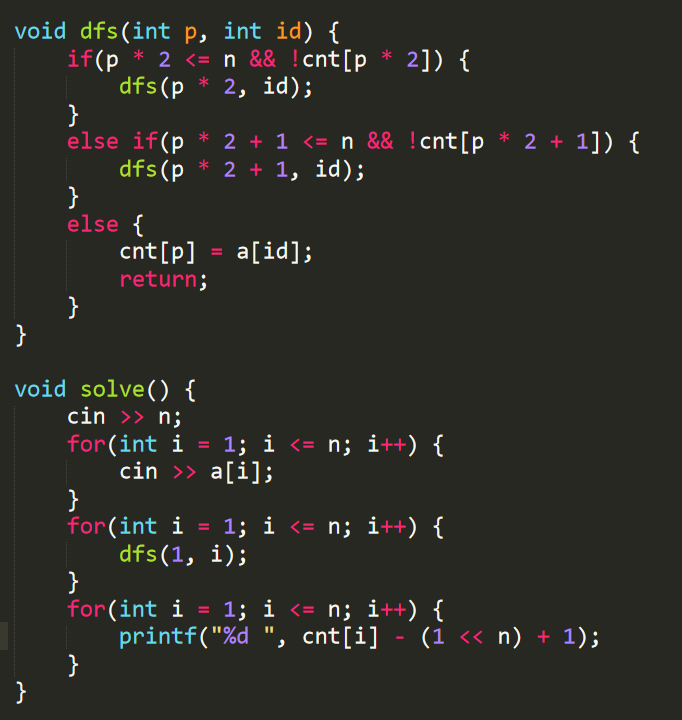展开全文• 线索二叉树后序线索化，后序遍历输出 一.写码思路 我们要进行后序遍历的化，需要记录每个结点的 Parent 结点，我之前觉得可能不需要这个，直接用前驱就行了，但是后序遍历结点的前驱不一定是他的 Parent 结点。 ...

## 一.写码思路

1. 我们要进行后序遍历的化，需要记录每个结点的 Parent 结点，我之前觉得可能不需要这个，直接用前驱就行了，但是后序遍历结点的前驱不一定是他的 Parent 结点。
例如下面这个例子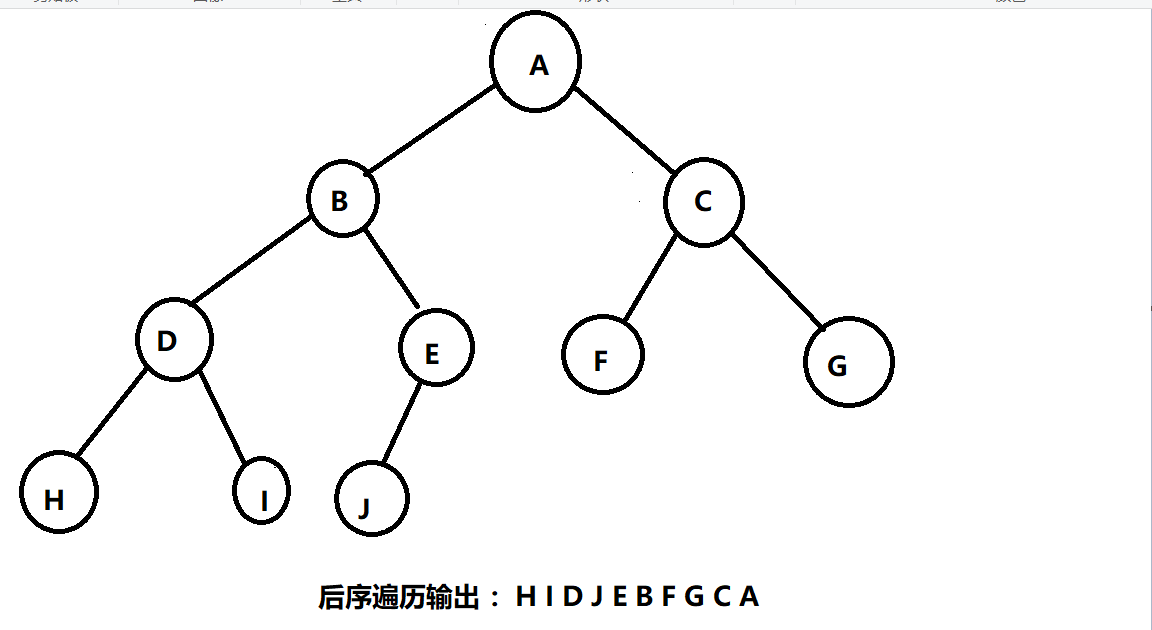B的Parent结点是A，但他的前驱是E
因此我们这个结点设定为如下
template<class T>
struct TreeNode
{
T Data;
TreeNode* Lkid;
TreeNode* Rkid;
TreeNode* Parent;	       //指向该节点的父母节点
int Ltag;
int Rtag;
};


Ltag和Rtag记录是否有孩子，并定义

#define Link	0	//表示该节点有孩子节点


①我们可以用一个类来封装这个二叉树，所以要进行构造，析构等函数
析构的时候我们可以用一个栈来储存这些结点，最后在析构的时候 delete 掉

②由于后序遍历的时候我们还要记录上一个访问过的结点，方便让结点指向他的前驱
我们设定为Pre_Node
然而这个 Pre_Node 在遍历的时候要一直记录上一个访问的结点，所以我们把他设为私有类

因此我们的类中就有这些成员

template<class T>
{
private:
TreeNode<T>* Pre_Node;
TreeNode<T>* Tree_Node_Stack[maxsize];

public:
TreeNode<T>* Tree;   //也可以写一个函数将这个成员数据 return 出来 ；其实也可以不把这个数据封装起来，直接在主函数设定一个Tree结点也完全ok的
void PostOrder(TreeNode<T>* &Tree);          //后序遍历
};


## 二.写准备遍历前的代码

1. 创造二叉树函数
//创造二叉树
template<class T>
{
char Data;
cin >> Data;
if (Data =='#')
Tree = NULL;
else
{
Tree = new TreeNode<T>;             //记得 delete 掉
Tree->Parent = Parent_Node;        //指向父母节点
Tree->Data = Data;
}
}


这个函数中 Tree 是根节点，由于在后面的函数中我们还要使用这个变化后的根节点，也就是说在之后的函数中，只要用到Tree，那他指的就是根节点，所以我们将这个Tree设为引用，不然在这个构造函数后，我们没有记录根节点是谁。
其次还要设定一个Parent_Node，用来记录父结点，用来连接父节点和孩子结点。我们将这个结点赋值给每个结点的 Parent 结点，递归的时候再将上一层的结点设定为 Parent_Node，这样这个结点在递归的时候就能赋值给他孩子结点的 Parent 结点。(注意 Parent_Node 和 Parent 结点不要弄混，Parent_Node只是个传值的，Parent指向每个结点的父节点指针，作用相当于左 ，右孩子指针）

2.构造函数

//初始化二叉树
template<class T>
Pre_Node(NULL)
{

}


3.析构函数

//析构二叉树
template<class T>
{
while (!Tree_stack.empty())
{
Tree_stack.pop();
}
}


我们在后面的后序遍历的时候，每遍历一个结点，我们就入栈一个结点，最后用上述的循环一个一个 delete。

## 三.后序线索化二叉树

我们遍历线索化的顺序是 左 右 根
所以先递归左孩子，再递归右孩子
最后来线索化根

template<class T>
{
if (Tree == NULL)
return;

if (Tree->Lkid == NULL)		        //根
{
Tree->Lkid = Pre_Node;
}
if (Pre_Node != NULL && Pre_Node->Rkid == NULL)
{
Pre_Node->Rkid = Tree;
}

Pre_Node = Tree;
}

1. 递归出口
当遍历的结点是NULL的时候，结束

2. 左孩子为空的时候
左指针指向前驱，就是我们再类里面定义的 Pre_Node

3.右孩子是难点
右孩子为空的时候，要指向他的后继结点
但是这个时候我们这个结点不好指向他的后继结点
只有这一层递归结束以后，我们才能来到这个结点的后继
如：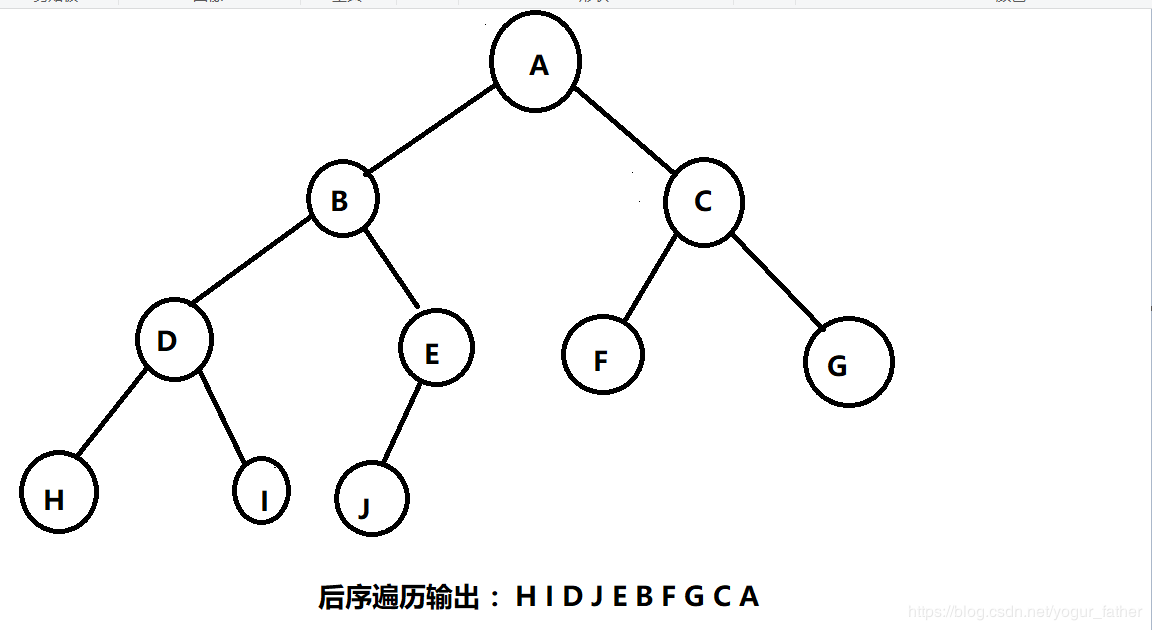D 结点的后继是 J，但这个时候 D 与 J 无联系，只有这一层递归结束，我们才能来到J结点
因此我们需要将当前结点设定为 Pre_Node,就是 Pre_Node = Tree;
这样我们来到 J 结点时，他的前一个结点的右孩子为空的话，就让他指向当前结点，也就是 Pre_Node的后继，再把右标识设定好。
再把当前节点设定为 Pre_Node。
如D是 Pre_Node，它指向当前结点，也就是 D 指向了 J。

## 四.后序遍历线索二叉树

后序遍历也是按照 左 右 中
所以我们先要找到最左边的结点
设定一个当前结点 Cur_Node
因此从根结点开始，一个一个判断，有左孩子，就往左。

	while (Cur_Node->Ltag == Link)//change,找到起始节点（在左树的最左边）
{
Cur_Node = Cur_Node->Lkid;
}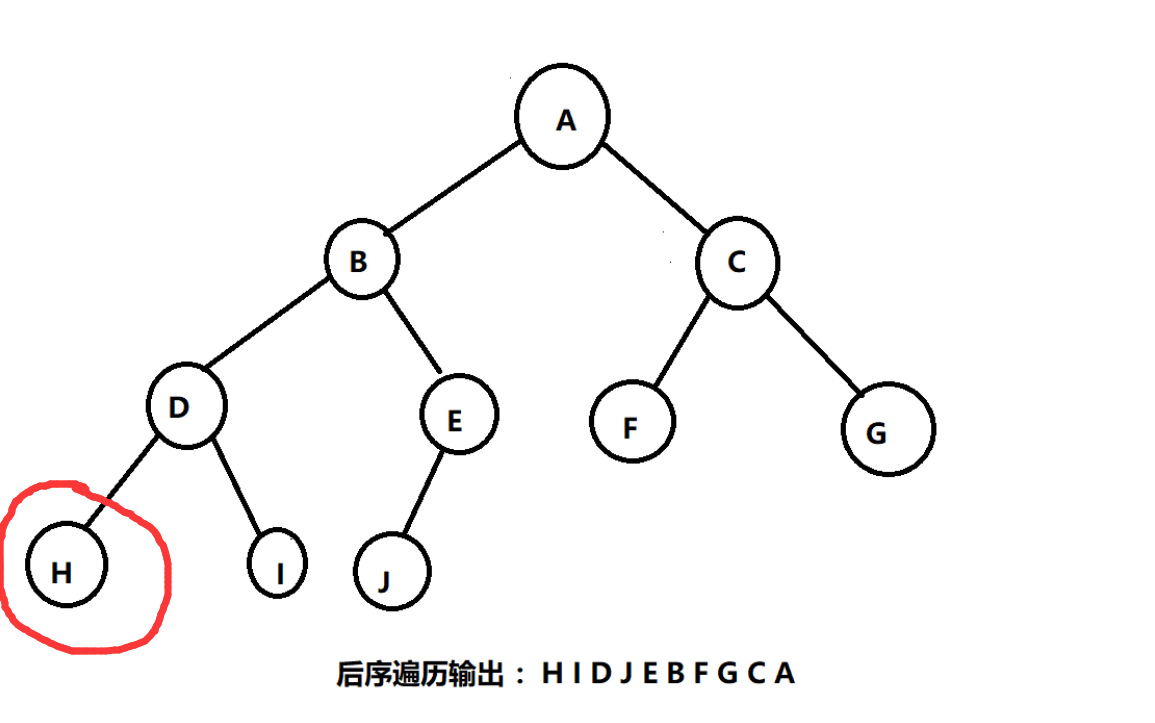（来到 H 结点）

之后访问他的后继
不过在访问后继之前先要把当前结点存到栈里面，方便后面析构
同时先输出当前结点

while (Cur_Node != NULL && Cur_Node->Rtag == Thread)//按线索找到次树节点
{
Tree_stack.push(Cur_Node)  ;
cout << Cur_Node->Data << " ";
Pre_Node = Cur_Node;			//每次访问节点后，PreNode更新
Cur_Node = Cur_Node->Rkid;
}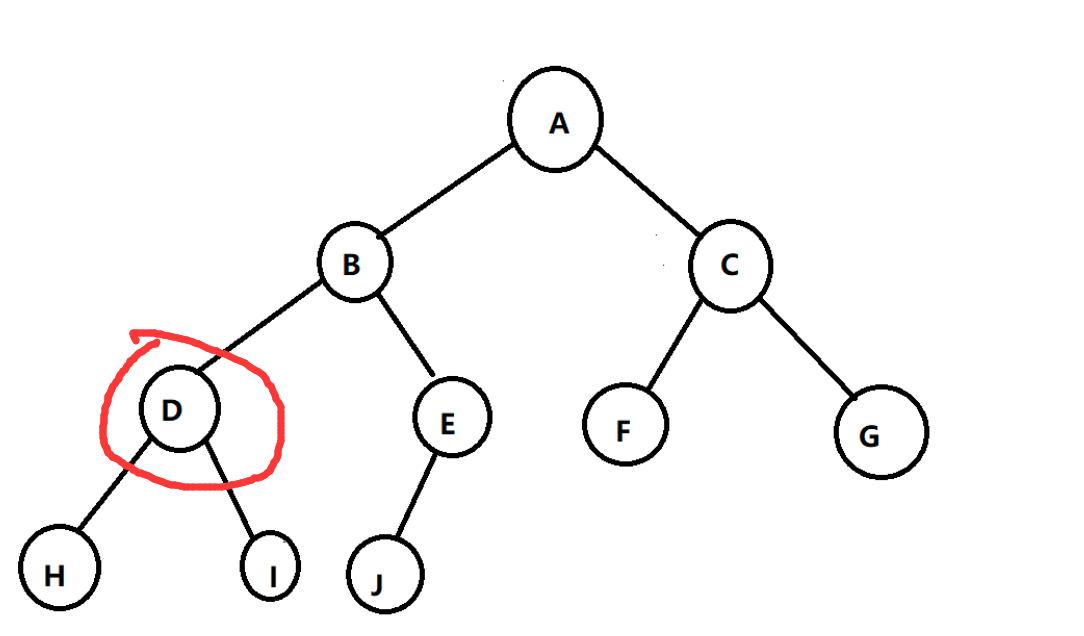（先到 I ,再来到 D 结点，因为 I 结点的后继是 D，但 D 的右指针又指向了 I ）

这样我们的当前节点就来到了子树的根节点
不过还需要判断 ， 判断条件就是 根节点的右指针是刚刚访问过的结点

while (Cur_Node != NULL && Cur_Node->Rkid == Pre_Node)//当前节点的右孩子节点刚好上次遍历，说明该子树只差根就遍历完成
{
Tree_stack.push(Cur_Node) ;
cout << Cur_Node->Data << " ";

if(Cur_Node==Tree)
return;
Pre_Node = Cur_Node;
Cur_Node = Cur_Node->Parent;		//访问子树后回到上一层
}


当然如果这个根节点是整个树的根节点，输出完之后结束程序
否则就指向这个子树根节点的父节点，为什么要指向父节点？因为D的右指针指向的是 I 结点
这样我们就来到了上一层树
最后记录访问过的结点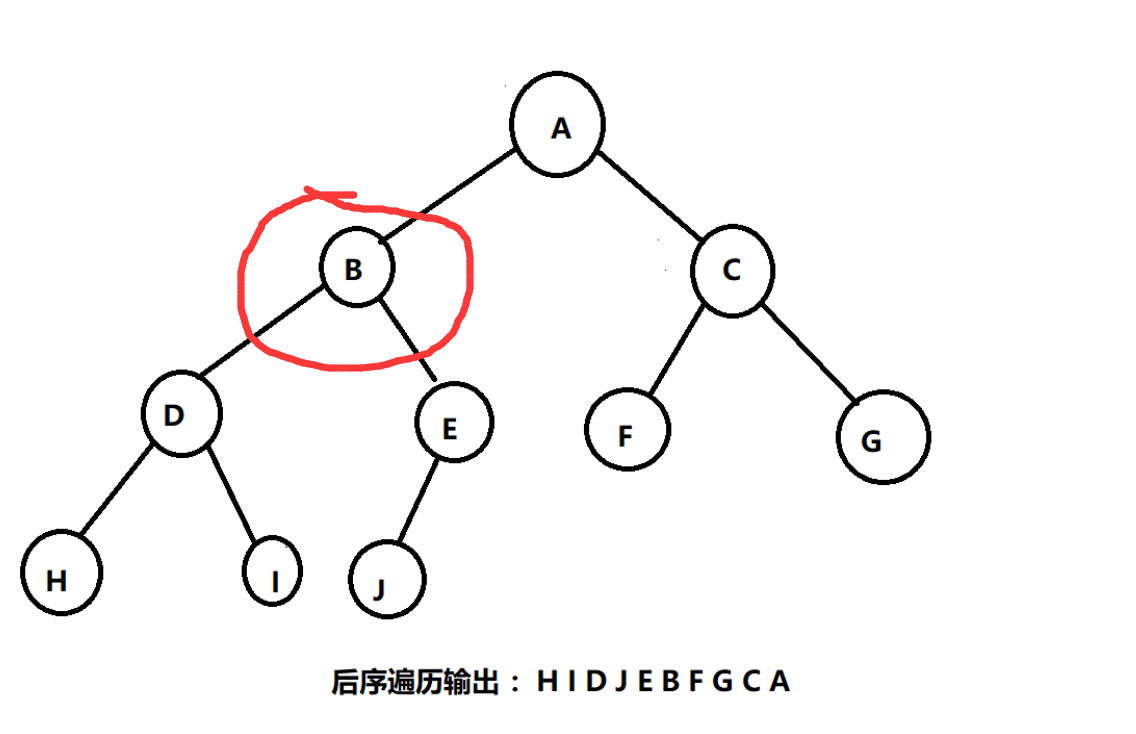（来到 B 结点，但不能输出）

这个时候我们要先遍历 B 结点右树的最左下结点，也就是 J

if (Cur_Node != NULL && Cur_Node->Rtag == Link)		//回到上一层后，先访问右孩子
{
Cur_Node = Cur_Node->Rkid;
}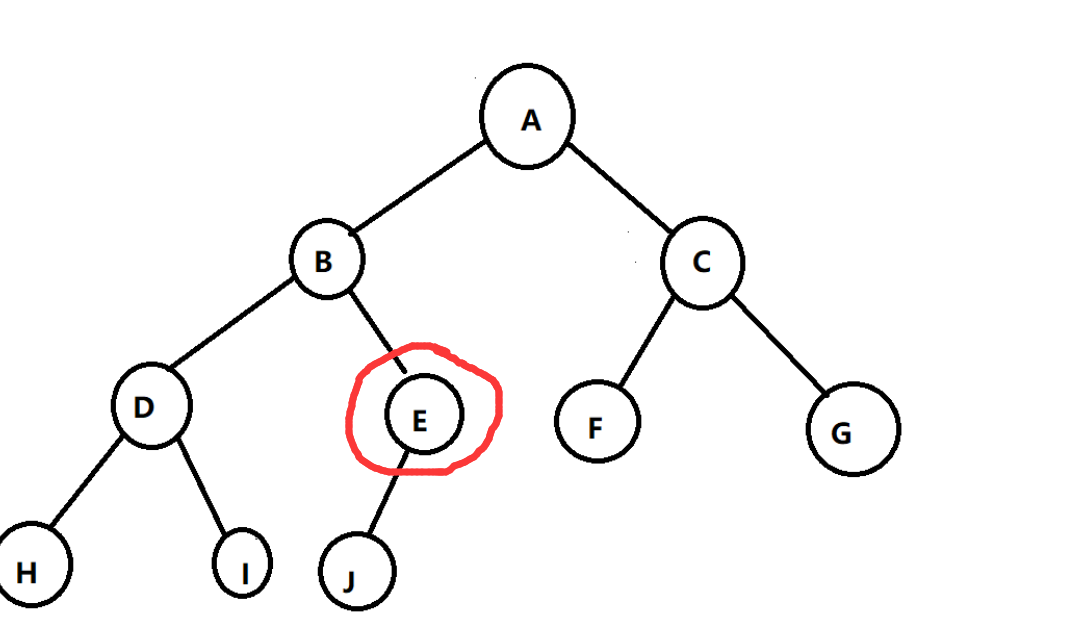但其实我们这个时候先到的是 E 结点，但是这一次最外面的循环就结束了，再从开头继续执行程序
记得我们循环开始的程序是什么吗？ 就是找到他最左下的结点，也就是 J

这样所有的结点就连起来了

这一部分的完整代码如下：

  //后序遍历二叉树
template<class T>
{
TreeNode<T> *Cur_Node = Tree;
Pre_Node = NULL;
while (Cur_Node != NULL)
{
{
Cur_Node = Cur_Node->Lkid;
}

while (Cur_Node != NULL && Cur_Node->Rtag == Thread)//按线索找到次树节点
{
Tree_stack.push(Cur_Node)  ;
cout << Cur_Node->Data << " ";
Pre_Node = Cur_Node;			//每次访问节点后，PreNode更新
Cur_Node = Cur_Node->Rkid;
}

while (Cur_Node != NULL && Cur_Node->Rkid == Pre_Node)//当前节点的右孩子节点刚好上次遍历，说明该子树只差根就遍历完成
{
Tree_stack.push(Cur_Node) ;
cout << Cur_Node->Data << " ";

if(Cur_Node==Tree)
return;
Pre_Node = Cur_Node;
Cur_Node = Cur_Node->Parent;		//访问子树后回到上一层
}

if (Cur_Node != NULL && Cur_Node->Rtag == Link)		//回到上一层后，先访问右孩子
{
Cur_Node = Cur_Node->Rkid;
}
}
}


## 五.完整代码

#include<iostream>
#include<stack>
using namespace std;

template<class T>
struct TreeNode
{
T Data;
TreeNode* Lkid;
TreeNode* Rkid;
TreeNode* Parent;	       //指向该节点的父母节点
int Ltag;
int Rtag;
};

template<class T>
{
private:
TreeNode<T>* Pre_Node;
stack<TreeNode<T>*> Tree_stack;

public:
TreeNode<T>* Tree;   //也可以写一个函数将这个成员数据 return 出来
void PostOrder(TreeNode<T>* &Tree);          //后序遍历
};

//创造二叉树
template<class T>
{
char Data;
cin >> Data;
if (Data =='#')
Tree = NULL;
else
{
Tree = new TreeNode<T>;
Tree->Parent = Parent_Node;        //指向父母节点
Tree->Data = Data;
}
}

//初始化二叉树
template<class T>
Pre_Node(NULL)
{

}

//析构二叉树
template<class T>
{
while (!Tree_stack.empty())
{
Tree_stack.pop();
}
}

//后序线索化二叉树
template<class T>
{
if (Tree == NULL)
return;

if (Tree->Lkid == NULL)		        //根
{
Tree->Lkid = Pre_Node;
}
if (Pre_Node != NULL && Pre_Node->Rkid == NULL)
{
Pre_Node->Rkid = Tree;
}

Pre_Node = Tree;
}

//后序遍历二叉树
template<class T>
{
TreeNode<T> *Cur_Node = Tree;
Pre_Node = NULL;
while (Cur_Node != NULL)
{
{
Cur_Node = Cur_Node->Lkid;
}

while (Cur_Node != NULL && Cur_Node->Rtag == Thread)//按线索找到次树节点
{
Tree_stack.push(Cur_Node)  ;
cout << Cur_Node->Data << " ";
Pre_Node = Cur_Node;			//每次访问节点后，PreNode更新
Cur_Node = Cur_Node->Rkid;
}

while (Cur_Node != NULL && Cur_Node->Rkid == Pre_Node)//当前节点的右孩子节点刚好上次遍历，说明该子树只差根就遍历完成
{
Tree_stack.push(Cur_Node) ;
cout << Cur_Node->Data << " ";

if(Cur_Node==Tree)
return;
Pre_Node = Cur_Node;
Cur_Node = Cur_Node->Parent;		//访问子树后回到上一层
}

if (Cur_Node != NULL && Cur_Node->Rtag == Link)		//回到上一层后，先访问右孩子
{
Cur_Node = Cur_Node->Rkid;
}
}
}

int main()
{
TreeNode <char> *parent;
MyTree.PostOrder(MyTree.Tree);
return 0;
}


## 六.测试数据

就用上面的例子来吧
abdh##i##ej###cf##g##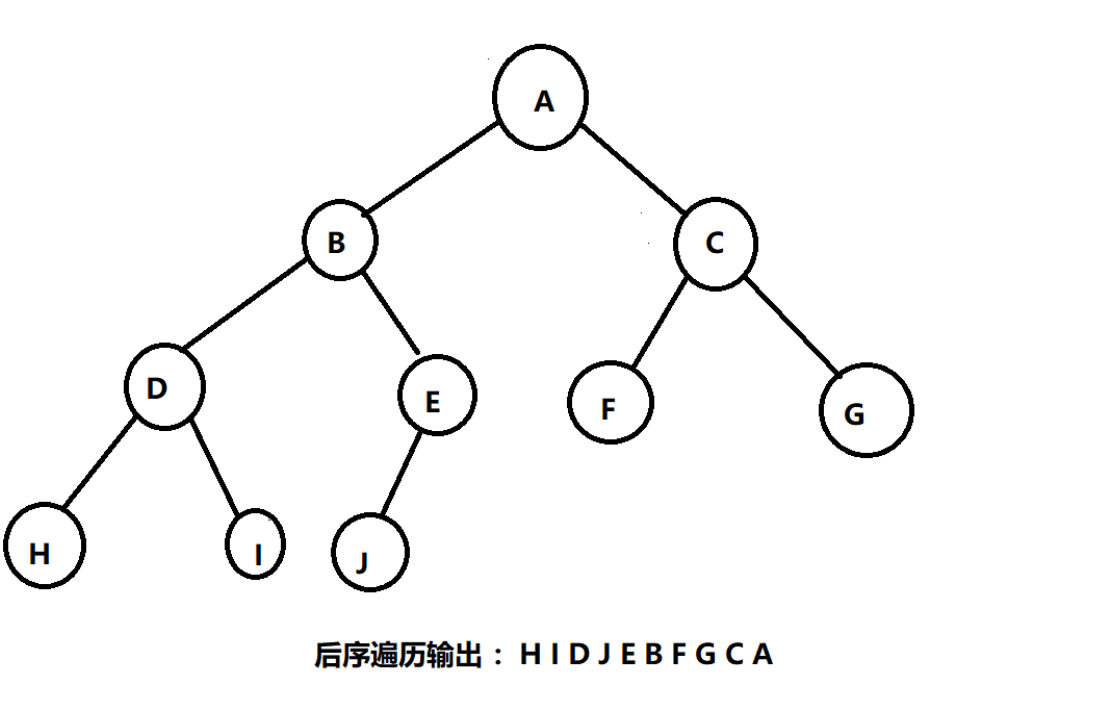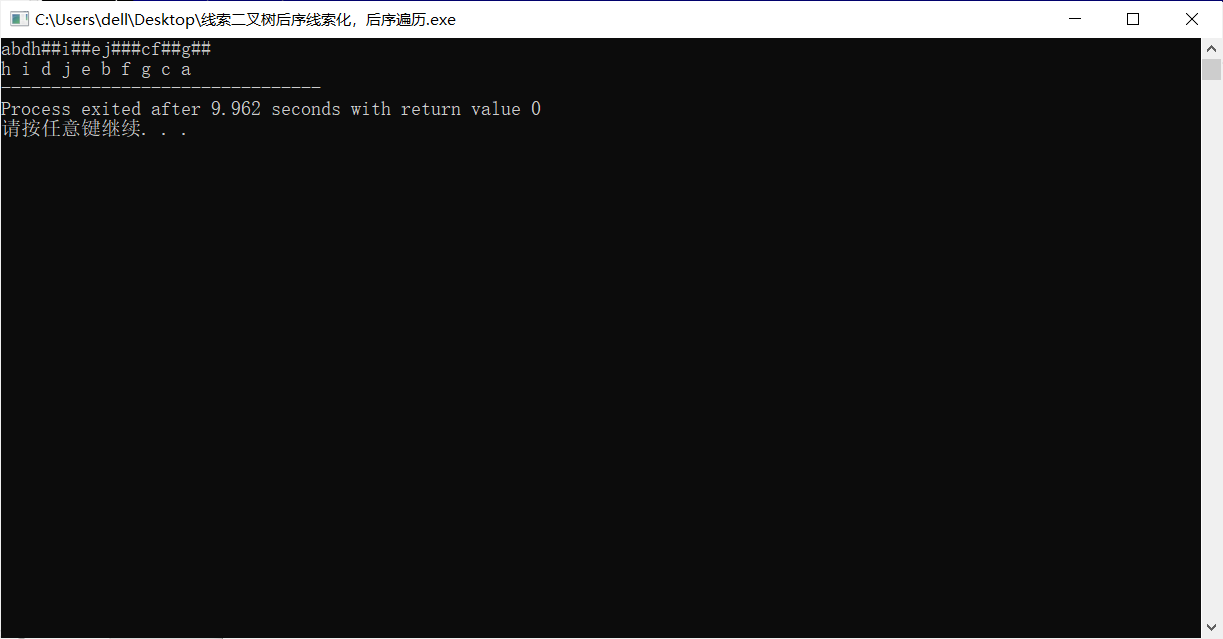再找个新例子，简单一点
12##34###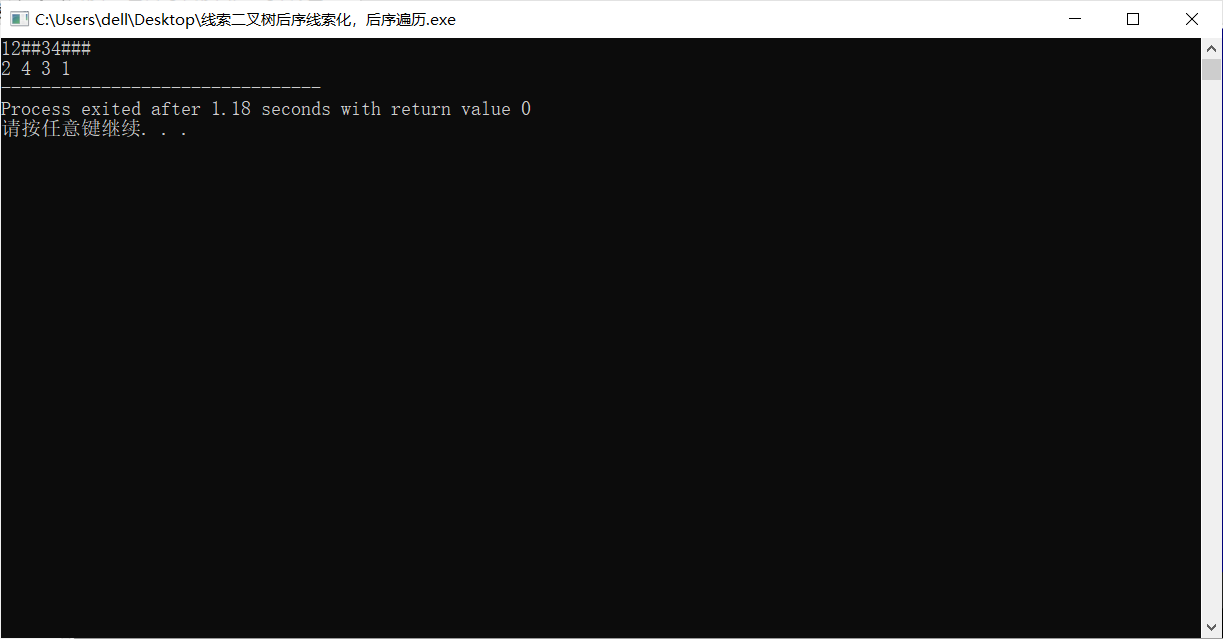到这里我们的后序遍历就完成了，希望能帮到你
反正我之前是把这个理解了好久

## 那之后能点个赞就更好了！！！

展开全文二叉树 数据结构 指针
• 数据结构C++二叉链表的先序遍历、中序遍历和后序遍历实现数据结构
• //后序遍历的最后一个结点为根结点 cout << node->elem;//输出当前结点 int rootIndex = 0; for (; rootIndex ; rootIndex++)//确定根结点位置 { if (inorder[rootIndex] == *(aftorder + length - 1))//中序...
• \n后序遍历二叉树： " ); PostOrderTraverse(T); system( " pause " ); return 0 ; }  解决思想：小生用的是递归创建二叉树，递归遍历二叉树，因为使用递归会比较简洁。（主要就是递归啦）。 PS：如若...
• printf("广义表表示的二叉树的输出：\n"); ListBinTree(t); printf("\n二叉树的前序遍历结果为：\n"); preorder(t); printf("\n二叉树的中序遍历结果为：\n"); inorder(t); printf("\n二叉树的后序便利...数据结构
• 输入后序遍历，中序遍历的结果。输出层次遍历的结果。 import java.util.Scanner; public class Main { public static void main(String[] args) { Scanner sc = new Scanner(System.in); String hx =sc.next();...算法 深度优先 数据结构
• 首先我们从两个方面讲解二叉树的后序遍历(递归+迭代) 一.二叉树的后序遍历.(递归) 思想: 首先我们从二叉树的根节点开始先遍历其左孩子,①接着同样继续遍历其左孩子的左孩子,直到某个左孩子节点的左孩子为NULL时,...c语言 数据结构 算法 链表 指针
• 给出一棵二叉树，分别输出先序、中序、后序遍历结果。 输入 第1行：结点数n(1 以下若干行,每行3个整数，分别表示父结点、左孩子、右孩子。若没有孩子，对应的整数为0. 输出 第1行：树根 第2行：先序遍历结果，数字间...二叉树
• 解题思路：不管是左右子树还是整棵树，后序遍历的最后一个元素就是根节点，然后从中序遍历中找到根节点在中序遍历的位置，并且记录左子树元素的个数。中序遍历中根节点以前的位左子树，以后的位右子树，然后递归遍...
• 剑指Offer(Python多种思路实现):二叉搜索树的后序遍历序列 面试33题： 题：二叉搜索树的后序遍历序列 题目：输入一个整数数组，判断该数组是不是某二叉搜索树的后序遍历的结果。如果是则输出Yes,否则输出No。假设...
• 根据先序遍历和中序遍历输出后序遍历 #include <stdio.h> #include <stdlib.h> char Pre, In; typedef struct Node { char Data; struct Node* Left; struct Node* Right; }*Tree; Tree Build...二叉树 数据结构 c语言
• 二叉树中已知中序遍历和前序遍历（后序遍历），求后序遍历（前序遍历）（C++）二叉树 c++
• 此文档所描述的内容是树的先序、中序、后序遍历算法，由C和C++混合编写的代码
• 输入一个整数数组，判断该数组是不是某二叉搜索树的后序遍历结果。如果是则返回 true，否则返回 false。假设输入的数组的任意两个数字都互不相同。 参考以下这颗二叉搜索树： 5 / \ 2 6 / \ 1 3 示例 1： 输入:...
• 输入一个整数数组，判断该数组是不是某二叉搜索树的后序遍历的结果。如果是则输出Yes,否则输出No。假设输入的数组的任意两个数字都互不相同。 解法一：递归法 # -*- coding:utf-8 -*- class Solution: def ...
• 后序遍历和中序遍历输出层序遍历C++ 给定一棵二叉树的后序遍历和中序遍历，请你输出其层序遍历的序列。这里假设键值都是互不相等的正整数。 输入格式： 输入第一行给出一个正整数N（≤30），是二叉树中结点的个数...c++ 二叉树 队列
• 较难较易混淆的二叉树部分经典例题，不会的童鞋~~二叉树
• 1）递归序，3次见面，第一次见面打印叫先序遍历，第二次见面打印叫中序遍历，第三次见面打印叫后序遍历 2）非递归实现中，先序和后序是相反的，但是中序遍历挺难理解，但是核心思想也就是先去左树，再打印头，然后再...二叉树 先序遍历 二叉树非递归遍历...